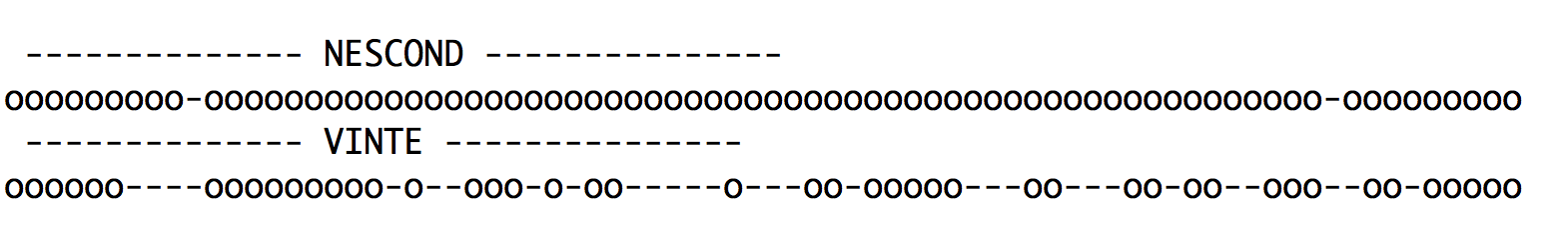# VINTE

## A theorem prover for Lewis' logics for counterfactual reasoning

A Prolog implementation of internal calculi for logic V and standard extensions

## Check the vaildity of a conditional formula

#### Do NOT use capital letters for propositional variables. Use:

• false for bottom
• true for top
• ^ for conjunction
• ? for disjunction
• - for negation
• -> for material implication
• => for conditional implication
• < for comparative plausibility

#### Examples:

• CPA: (a < a ? b) ? (b < a ? b)
• TR: (a < b) ^ (b < c) -> (a < c)
• CO: (a < b) ? (b < a)

## Prolog Source Code

Conditional Logic Axioms Characterizing Axiom Prolog Source Code
V CPR, CPA, TR, CO - code
VN CPR, CPA, TR, CO, N -(false < true) code
VT CPR, CPA, TR, CO, N, T (false < -a) -> a code
VW CPR, CPA, TR, CO, N, T, W a -> (a < true) code
VC CPR, CPA, TR, CO, N, T, W, C (a < true) -> a code
VA CPR, CPA, TR, CO, A1, A2
 (a < b) -> (false < -(a < b)) -(a < b) -> (false < (a < b))
code
VNA CPR, CPA, TR, CO, N, A1, A2
 -(false < true) (a < b) -> (false < -(a < b)) -(a < b) -> (false < (a < b))
code

## Experiments

We have compared VINTE with three theorem provers for conditional logics:

• NESCOND - nested sequents for conditional logics
• GoalDUCK - goal-directed proof search for conditional logics
• CondLean - labelled sequent calculi for conditional logics

Performances of VINTE are promising, as witnessed by the following results obtained by testing the four provers over radomly genrated sequents.

The following tables show the number of timeouts over sequents with 100 formulas and a level of nesting 20 for logic V:

Theorem prover Time limit 5ms Time limit 1s
VINTE (for V) 45 0
CondLean 173 141
GoalDUCK 136 133
NESCOND 479 0

We have tested VINTE over 76 valid formulas, and compared its performance with those of NESCOND implementing internal calculi for conditional logics.

The following figure shows successes provided by the two provers within 1 ms (° are successes, - are timeouts):In the next figure we show successes with a time limit of 3 minutes:Valid formulas are obtained by translating modal logic K valid formulas provided by Heuerding in conditional formulas of conditional logic CK. NESCOND is faster, since it is specifically tailored for CK, but performance of VINTE are promising.

## Prolog code

VINTE over random sequents: V - VN - VT - VW - VC - VA - VNA
VINTE vs NESCOND over valid formulas code